# Python How to calculate the quantity by investment and leverage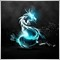88

Hi, I am using python to build an expert advisor. Right now I have a function which calculate the quantity by the investment but dosent take into account the leverage so how I calculate the leverage?

Here is my function:

```def qty_buy(symbol, investment, df):
try:
price = get_price(df)
symbol_info = mt5.symbol_info(symbol)._asdict()
digits = symbol_info['digits']
min_vol = symbol_info['volume_min']
max_vol = symbol_info['volume_max']
vol_steps = symbol_info['volume_step']
tcsv_min = (tcs * price) * min_vol
tcsv_max = (tcs * price) * max_vol
pos_qty = []
qty_value = 0
if investment < tcsv_min:
qty = 0
##        elif (investment / price) < min_vol:
##            qty = 0
elif investment > tcsv_max:
qty = max_vol
##        elif (investment / price) > max_vol:
##            qty = max_vol
else:
for i in np.arange((tcsv_min), (tcsv_max), (vol_steps * price)):
pos_qty.append(i)
if len(pos_qty) > 0:
##                qty = find_nearest(pos_qty, (investment / price))
qty_value = find_nearest(pos_qty, (investment))
else:
qty_value = 0
j = 0
while j <= int(qty_value):
if qty_value > (investment):
qty_value = qty_value - (vol_steps * price)
else:
break
j += 1
qty = qty_value / price
if qty < min_vol:
qty = 0
if '#' in symbol and qty < 50:
qty = 0
##        print(qty)
##        sys.exit()
return qty
except Exception as e:
print("Error code =",mt5.last_error())
print(e, 'error ', traceback.format_exc())
pass```

I am taking an investment of 10% of the account_info.margin_free  for testings. I need to add the leverage which is 1:30 with my broker so how do I do this? whats the formula?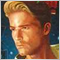962

Leverage is irrelavant.

My understanding of leverage so far, please correct me if I am wrong !!

William Roeder, 2017.09.11 15:30

1. You don't pay 360 to the broker, he impounds it and releases it when you close the trade.
2. As you said, leverage can be dangerous. You shouldn't be even thinking about it. You should be thinking about your risk on a trade. The leverage just allows it. Never risk more than a small percentage of your balance on any single trade.
3. In code: Risk depends on your initial stop loss, lot size, and the value of the pair.
• You place the stop where it needs to be - where the reason for the trade is no longer valid. E.g. trading a support bounce the stop goes below the support.
• Account Balance * percent/100 = RISK = OrderLots * (|OrderOpenPrice - OrderStopLoss| * DeltaPerLot + CommissionPerLot) (Note OOP-OSL includes the SPREAD, and DeltaPerLot is usually around \$10/pip but it takes account of the exchange rates of the pair vs. your account currency.)
• Do NOT use TickValue by itself - DeltaPerLot
• You must normalize lots properly and check against min and max.
• You must also check FreeMargin to avoid stop out
Most pairs are worth about \$10 per PIP. A \$5 risk with a (very small) 5 PIP SL is \$5/\$10/5=0.1 Lots maximum. Use a EA GUI such as the one for MT4: Indicators: 'Money Manager Graphic Tool' indicator by 'takycard' Forum - Page 588

I have found a way but it seems diferent instruments have diferent leverage so the new question is what in symbol info tell me the leverage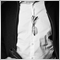268788

Thank you for the link but i solved the quantity calculation problem. The only problem remaining is how I find the symbol leverage from what is provided? Because in symbol_info there is no such information2687

https://www.mql5.com/en/docs/constants/environment_state/marketinfoconstants#enum_symbol_calc_mode962

Dragos Drimbe #:
Thank you for the link but i solved the quantity calculation problem. The only problem remaining is how I find the symbol leverage from what is provided? Because in symbol_info there is no such information

Strange enough, There is no leverage property at symbol level.88

Dominik Christian Egert #:

Thx but that is for MT5 language. Could be this for Python? https://www.mql5.com/en/docs/integration/python_metatrader5/mt5ordercalcmargin_py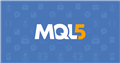Documentation on MQL5: Integration / MetaTrader for Python / order_calc_margin
• www.mql5.com
order_calc_margin - MetaTrader for Python - Integration - MQL5 Reference - Reference on algorithmic/automated trading language for MetaTrader 5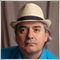11356

Dragos Drimbe #:Thx but that is for MT5 language. Could be this for Python? https://www.mql5.com/en/docs/integration/python_metatrader5/mt5ordercalcmargin_py

It is the same thing in python by using the "symbol_info" and I assume it is the "trade_calc_mode" property according to the example code in the documentation. The constants will be the same as the MQL5 documentation.2687

Enrique Dangeroux #:

Strange enough, There is no leverage property at symbol level.

Thats because leverage is the result of a calculation given by margin values given by the symbol.

Your account has a leverage value, but every symbol can define their own margin parameters, overwriting your accounts leverage.

If you understand the formulas, and you work with some symbols and brokers, you will find out how this works.

It took me quite some time to figure out. But once understood, it will be quite intuitive.88

It dosent seems to find a way to get the leverage for the symbol, I have tryied maket_book_get which return none and margin_calc which give something else and it seems no one else knows